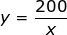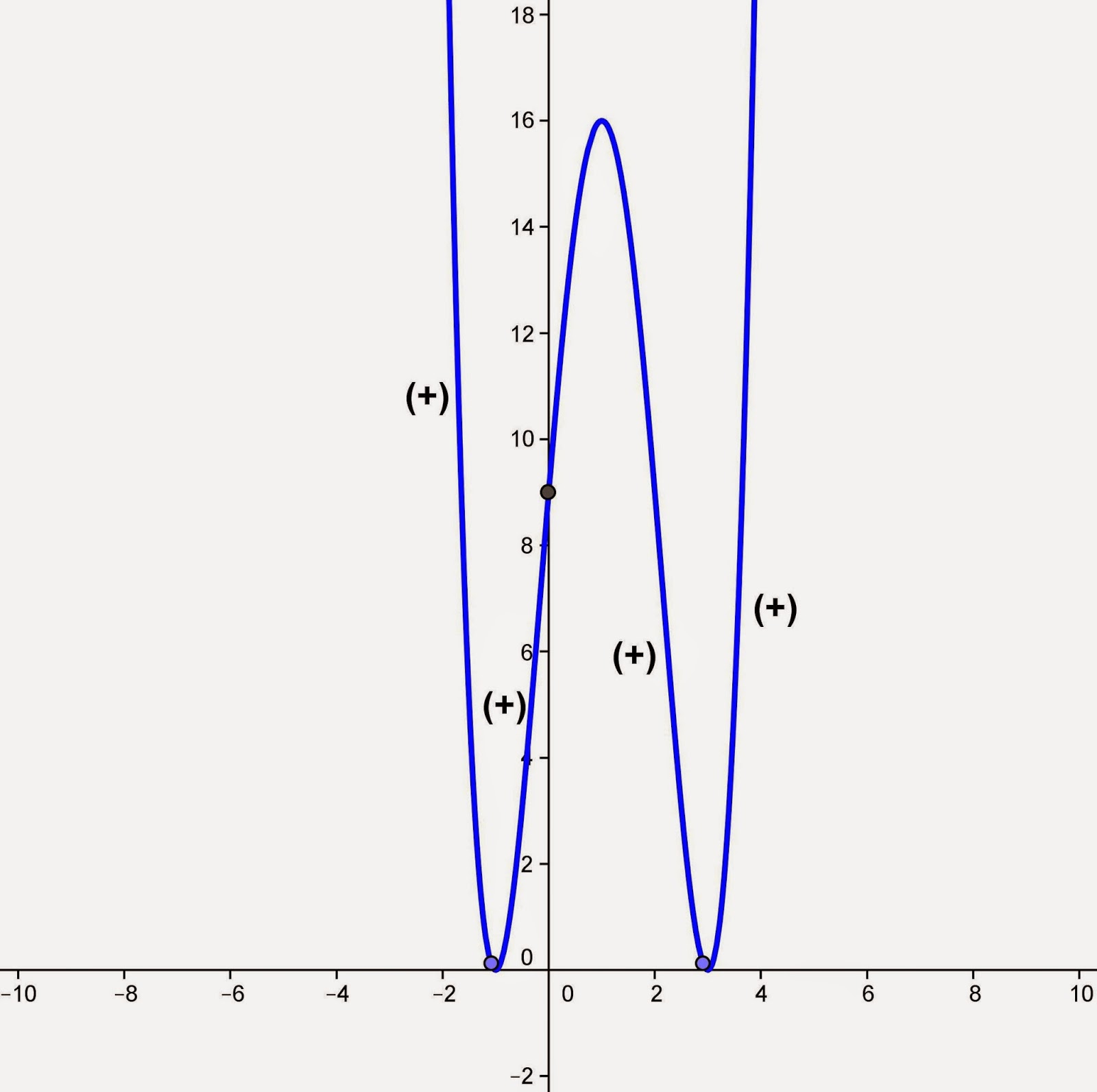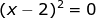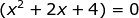## Sunday, November 30, 2014

### Money and Investment Problems, 5

Category: Algebra

"Published in Newark, California, USA"

In 1964, Rosario invested a sum of money in the Philippine Catsup Company which gave her a return of ₱ 200 at the end of the year. Had she invested the amount in the Manila Tiles Corporation which declared a dividend of ½ % more, she would have made ₱ 70 more with an investment of ₱ 2,000 less. How much did she invest in the Philippine Catsup Company?

Solution:

The given word problem is about money and investment problem in which the money invested, annual interest rate, and annual income are involved. Let's analyze the given word problem as follows:

Let x = be the amount of money invested at Philippine Catsup Company
y = be the annual interest rate at Philippine Catsup Company

If the word problem says "Rosario invested a sum of money in the Philippine Catsup Company which gave her a return of ₱ 200 at the end of the year.", then the working equation which is her annual income in the said company is

If the word problem says "Had she invested the amount in the Manila Tiles Corporation which declared a dividend of ½ % more, she would have made ₱ 70 more with an investment of ₱ 2,000 less.", then the working equation which is her annual income in the said company is

Next, use the two working equations in order to solve for the value of x and y which are the amount of money invested at Philippine Catsup Company and its annual interest rate, respectively.

ButHence, the above equation becomes

By using the completing the square method, the value of x which is the amount of money invested at Philippine Catsup Company is

We need to choose the positive sign since we want to get the amount of money invested at Philippine Catsup Company.

The annual interest rate at Philippine Catsup Company is

Therefore, Rosario invested ₱ 20,000 at Philippine Catsup Company with the annual interest rate of 1.0 %.

## Saturday, November 29, 2014

### Sketching the Graph of a Polynomial, 9

Category: Analytic Geometry

"Published in Vacaville, California, USA"

Sketch the graph of a polynomial:

Solution:

Consider the given equation above

Did you notice that the trinomial inside the parenthesis is factorable by the product of two binomials? Let's factor the above equation as follows

If we set y = 0, then the x-intercepts of the given equation are 3, and -1.

If we set x = 0, then the y-intercept of the given equation is

Since we now the x-intercept, then we can sketch the location or direction of a curve as follows

If x < -1, then y = (-)²(-)² = (+)
If -1 < x < 0, then y = (-)²(+)² = (+)
If 0 < x < 3, then y = (-)²(+)² = (+)
If x > 3, then y = (+)²(+)² = (+)

Here's the graph of a polynomial:Photo by Math Principles in Everyday Life

## Friday, November 28, 2014

### Sketching the Graph of a Polynomial, 8

Category: Analytic Geometry

"Published in Vacaville, California, USA"

Sketch the graph of a polynomial:

Solution:

Consider the given equation above

Did you notice that the given equation can be factored by grouping? Let's factor the above equation as follows

and then it is factorable by the difference of two cubes as follows

If we set y = 0, then we can solve for the value of x at each factors which are the x-intercepts.

For, the value of x is

For, the value of x is

Since the roots of the second factor are imaginary numbers, then we cannot accept those values. Because of this, the only x-intercept is 2.

If we set x = 0, the y-intercept of the given equation is

Since we now the x-intercept, then we can sketch the location or direction of a curve as follows

If x < 0, then y = (-)²(+) = (+)
If 0 < x < 2, then y = (-)²(+) = (+)
If x > 2, then y = (+)²(+) = (+)

Here's the graph of a polynomial:Photo by Math Principles in Everyday Life